# RECIPROCAL LATTICE a b 0 1 REAL LATTICE

• Slides: 21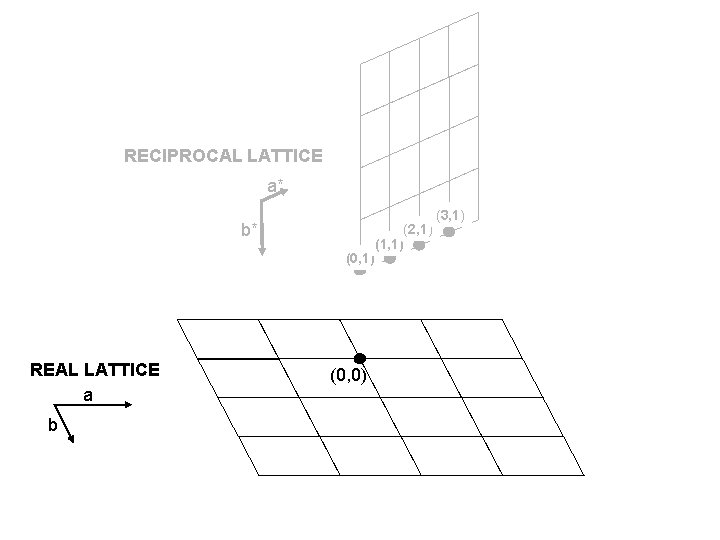RECIPROCAL LATTICE a* b* (0, 1) REAL LATTICE a b (0, 0) (2, 1) (1, 1) (3, 1)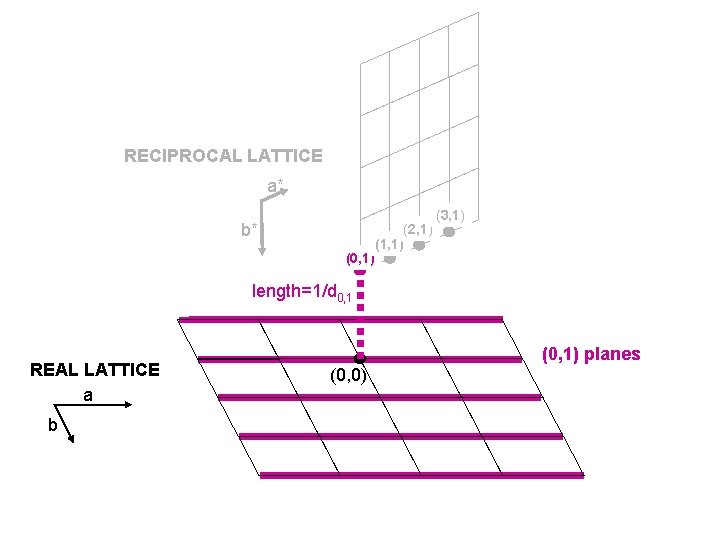RECIPROCAL LATTICE a* b* (0, 1) (2, 1) (1, 1) (3, 1) length=1/d 0, 1 REAL LATTICE a b (0, 1) planes (0, 0)RECIPROCAL LATTICE a* b* (0, 1) length=1/d 1, 1 REAL LATTICE a b (0, 0) (2, 1) (1, 1) (3, 1) length is longer than (0, 1) since spacing between (1, 1) planes is smaller. (1, 1) planes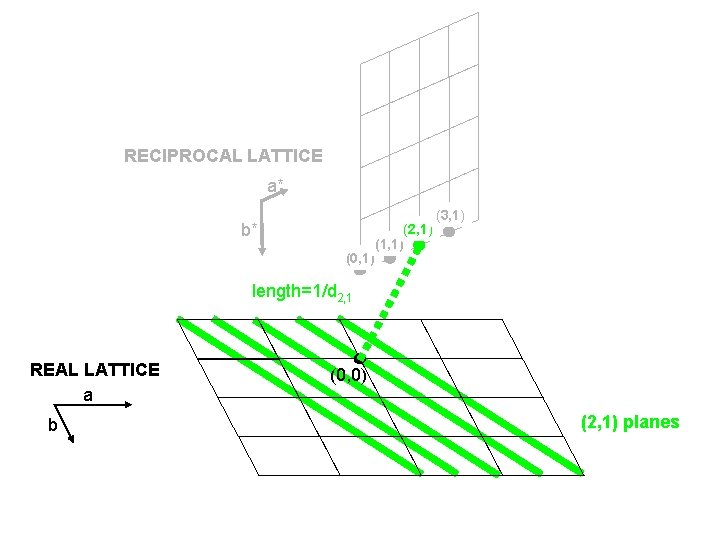RECIPROCAL LATTICE a* b* (0, 1) (2, 1) (1, 1) (3, 1) length=1/d 2, 1 REAL LATTICE a b (0, 0) (2, 1) planesRECIPROCAL LATTICE a* b* (0, 1) (2, 1) (1, 1) (3, 1) length=1/d 3, 1 REAL LATTICE a (0, 0) b (3, 1) planesRECIPROCAL LATTICE a* b* (0, 1) REAL LATTICE a b (2, 1) (1, 1) (3, 1) (0, 1) planes (0, 0) (1, 1) planes (2, 1) planes (3, 1) planesRECIPROCAL LATTICE a* (0, 2) b* (0, 1) (2, 1) (1, 1) (3, 1) length=1/d 0, 2 REAL LATTICE a b (0, 2) planes (0, 0)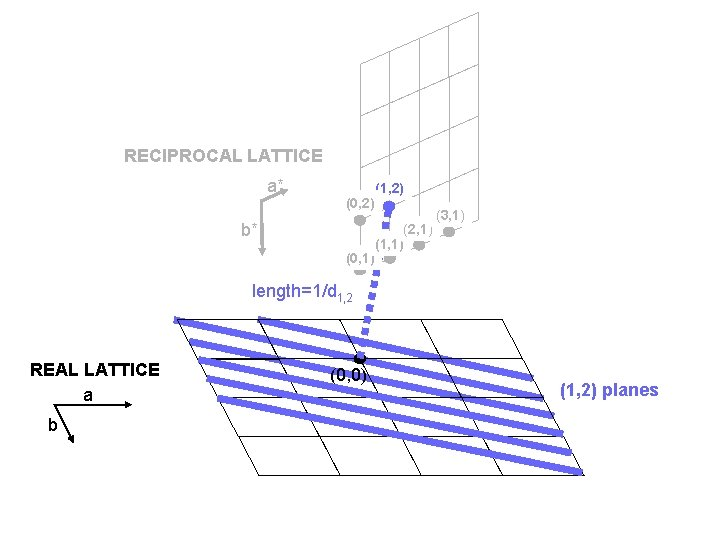RECIPROCAL LATTICE a* (0, 2) b* (0, 1) (1, 2) (2, 1) (1, 1) (3, 1) length=1/d 1, 2 REAL LATTICE a b (0, 0) (1, 2) planesRECIPROCAL LATTICE a* (0, 2) b* (0, 1) (2, 2) (1, 2) (2, 1) (1, 1) (3, 1) length=1/d 2, 2 REAL LATTICE a b (0, 0) (2, 2) planesRECIPROCAL LATTICE a* (0, 2) b* (0, 1) (2, 2) (1, 2) (2, 1) (1, 1) (3, 2) (3, 1) length=1/d 3, 2 REAL LATTICE a (0, 0) b (3, 2) planes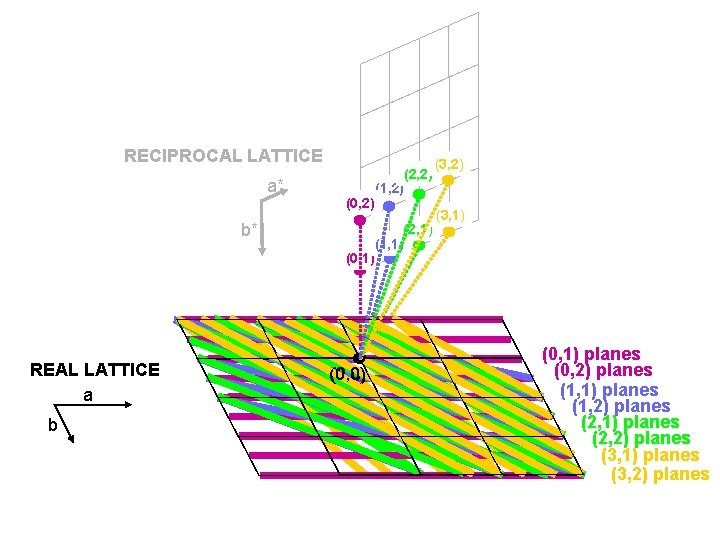RECIPROCAL LATTICE a* (0, 2) b* (0, 1) REAL LATTICE a b (0, 0) (2, 2) (1, 2) (2, 1) (1, 1) (3, 2) (3, 1) (0, 1) planes (0, 2) planes (1, 1) planes (1, 2) planes (2, 1) planes (2, 2) planes (3, 1) planes (3, 2) planes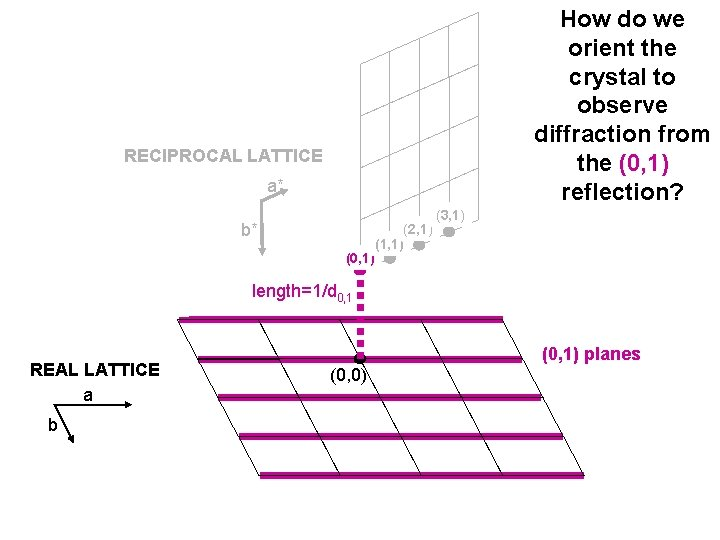How do we orient the crystal to observe diffraction from the (0, 1) reflection? RECIPROCAL LATTICE a* b* (0, 1) (2, 1) (1, 1) (3, 1) length=1/d 0, 1 REAL LATTICE a b (0, 1) planes (0, 0)q (0, 0) nl=2 dsinq Bragg condition-- upper beam has to be an integral number of wavelengths from the lower constructive interference. (0, 1) planes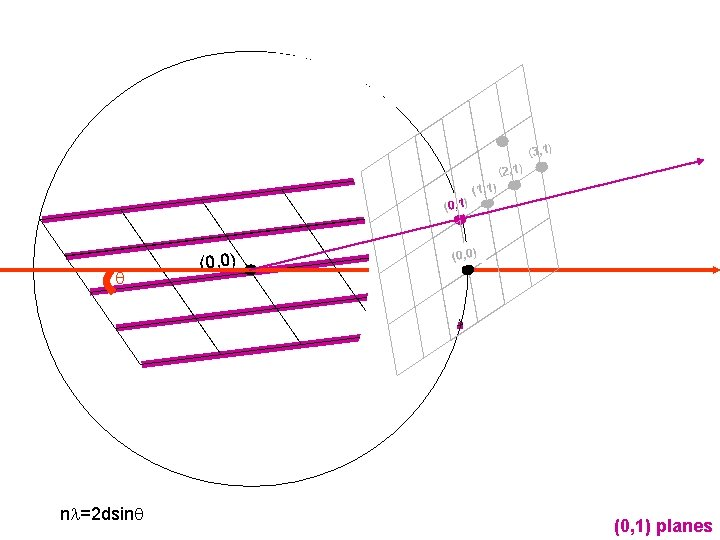(3, 1) (2, 1) (0, 1) q nl=2 dsinq (0, 0) (1, 1) (0, 0) (0, 1) planes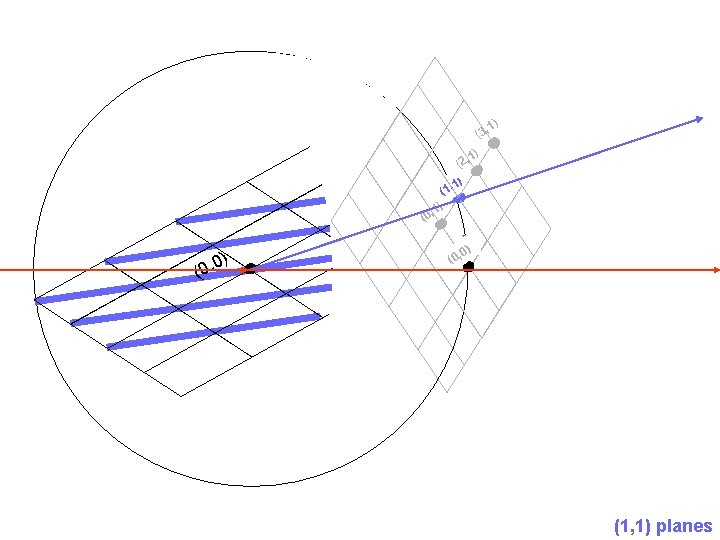(3, (0 (0 , 0) (1 , 1) (2, , 1) 1) 1) ) 0 (0, (1, 1) planes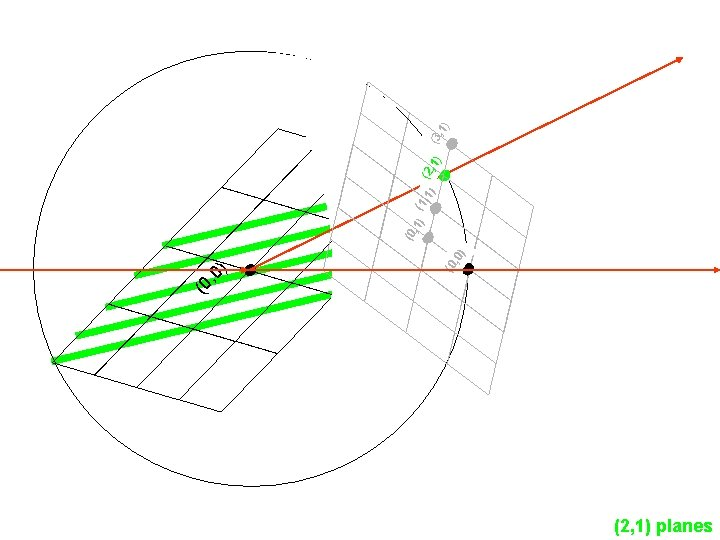(2, 1) planes ) (0 , 0 ) , 0 (0 ) (1, 1) (2, 1) , 1 (0 (3 , 1)